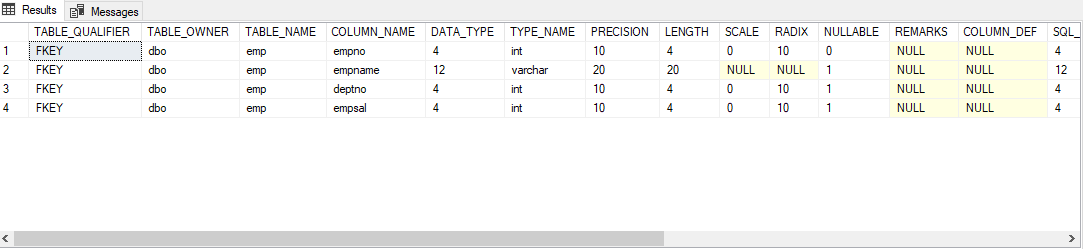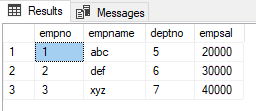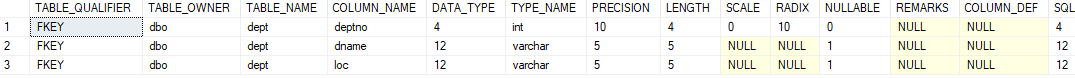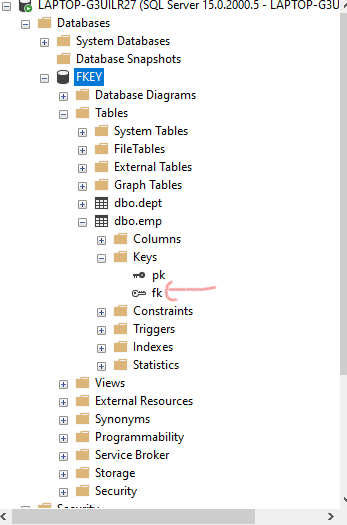# SQL Query to Drop Foreign Key Constraint Using ALTER Command

Here, we are going to see How to Drop a Foreign Key Constraint using ALTER Command(SQL Query) using  Microsoft SQL Server.

A Foreign key is an attribute in one table which takes references from another table where it acts as the primary key in that table. Also, the column acting as a foreign key should be present in both tables.

### Creating a new Database:

`CREATE DATABASE geeks;`

### Using the Database:

`USE geeks;`

### Table Definition:

We have the following emp table in our database :

```CREATE TABLE emp(
empno number(2) constraint pk primary key ,
empname varchar2(20),
deptno number(2),
empsal number(20));```

To verify table schema use the following query:

`EXEC SP_COLUMNS emp;`

Output:EMP TABLE SCHEMA

Use the below statement to add data to the emp table:

```INSERT INTO emp values(1,'abc',5,20000);
INSERT INTO emp values(2,'def',6,30000);
INSERT INTO emp values(3,'xyz',7,40000);```

Output:VALUES IN EMP TABLE

Now let’s write SQL Query to Drop Foreign Key Constraint Using ALTER Command. For that, we have to create another table called “DEPT”.

### Creating DEPT table

```CREATE TABLE dept(
deptno number(2) constraint pk2 primary key ,
dname varchar2(5),
loc varchar2(5));```

To check out the current table use the following statement:

`SELECT * FROM dept;`

Output:DEPT TABLE SCHEMA

### Adding Data to Dept Table:

Use the below statement to add data to the dept table:

```INSERT INTO dept values(5,'IT','hyd');
INSERT INTO dept values(6,'sales','bglr');
INSERT INTO dept values(7,'mgr','mumb');```

To check out the current table use the following statement:

`SELECT * FROM dept;`

### OutputDEPT TABLE VALUES

Here we have kept the DEPTNO column as common in both EMP and DEPT tables

```ALTER TABLE emp add constraint fk foreign key(deptno) references dept(deptno);
//ADDS FOREIGN KEY CONSTRAINT ON EMP TABLE```FOREIGN KEY “FK” HAS BEEN CREATED

### Dropping  Foreign Constraint:

`ALTER TABLE TABLE NAME drop constraint CONSTRAINT_NAME`

### Removing Foreign key Constraint from the table using ALTER:

`ALTER TABLE emp drop constraint fk;`

Output:Hence, in this way, we can Drop Foreign Key Constraint Using ALTER Command

Unlock the Power of Placement Preparation!
Feeling lost in OS, DBMS, CN, SQL, and DSA chaos? Our Complete Interview Preparation Course is the ultimate guide to conquer placements. Trusted by over 100,000+ geeks, this course is your roadmap to interview triumph.